CAT | Tech Mahindra Quants Questions

## Tech Mahindra Quants QuestionsJobs By Batch
Jobs By Location
Jobs By Degree
Jobs By Branch
IT Jobs
Internships
Govt. Jobs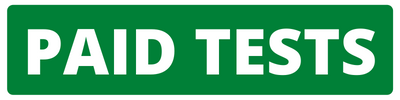• ##### Original Questions of IT Companies| All Topics### Quiz Begins Here

Q #1
:

#### Quants Question

Directions: These questions are based on the following graph which gives the profit percentages of three companies A, B and C for the six years from 1995 to 2000.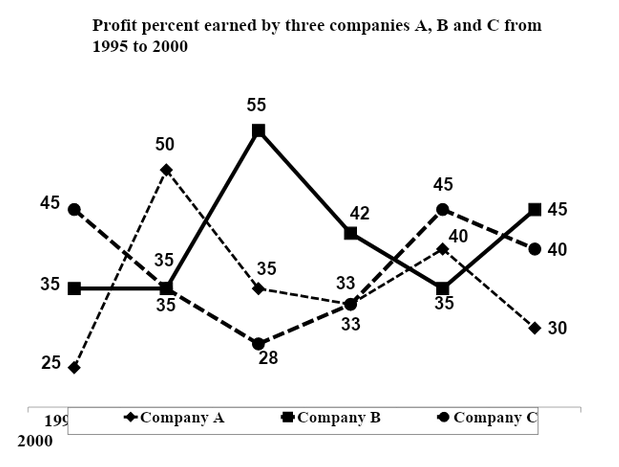The profit of a company is defined as the difference between the Income and Expenditure (where Income > Expenditure). The profit percent of a company is calculated with respect to the income.

If the expenditure of company C in 1995 was the same as the expenditure of company B in 2000 which was Rs.180 lakhs, what is the ratio of the income of company B in the year 2000 to that of company C in 1995?
+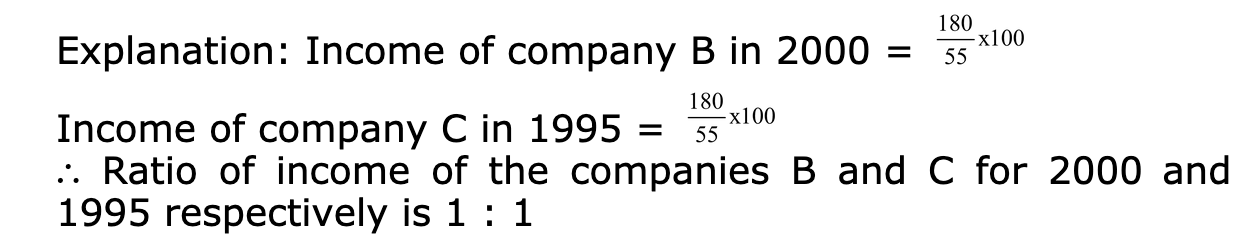Q #2
:

#### Quants Question

Directions: These questions are based on the bar graph, which gives the production and sales of trinkets in India, for the period 1991 to 1994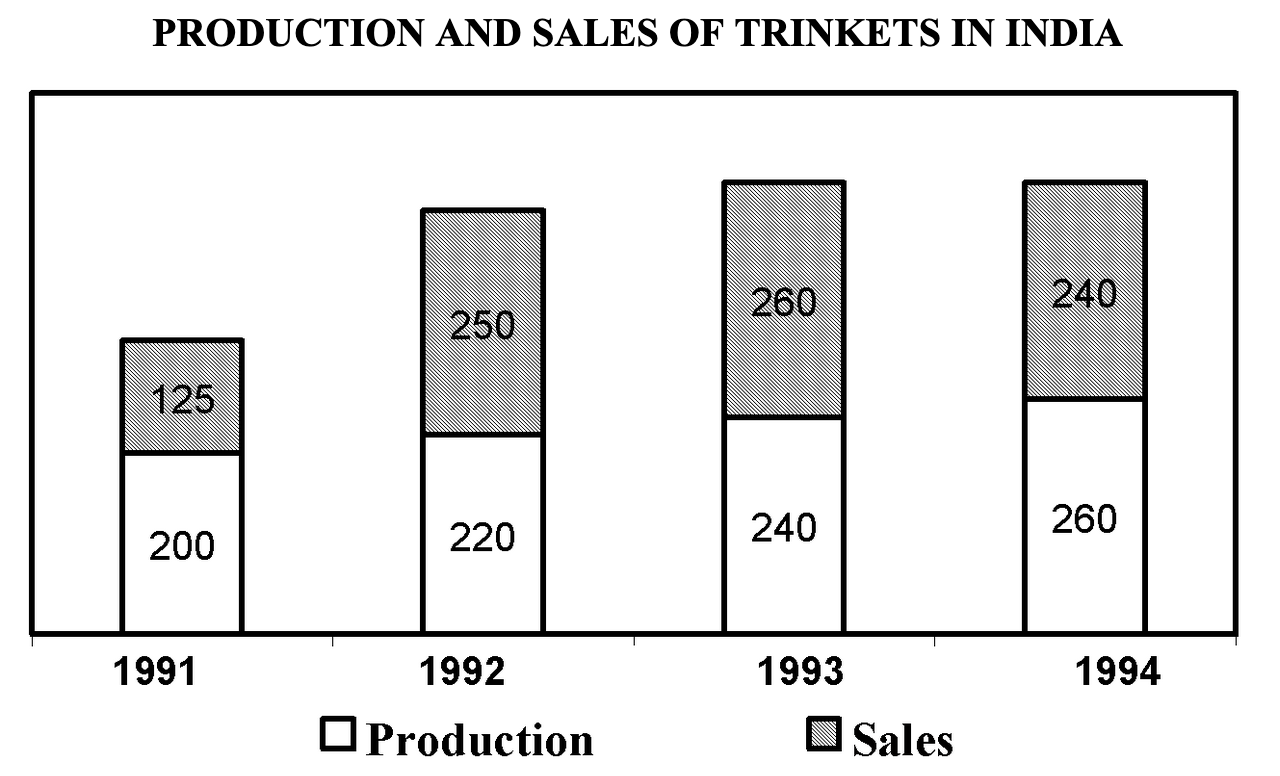If the excess/shortfall in production with respect to the sales in any year is exported/imported in the same year, then in 1993 the imports form what percentage of the sales?
+

Explanation: Imports in 1993 = 20/260 = 7.7%

Q #3
:

#### Quants Question

Directions: These questions are based on the following table, which gives the fatality and accident rates in coal mines in India for the period 1973 to 1989.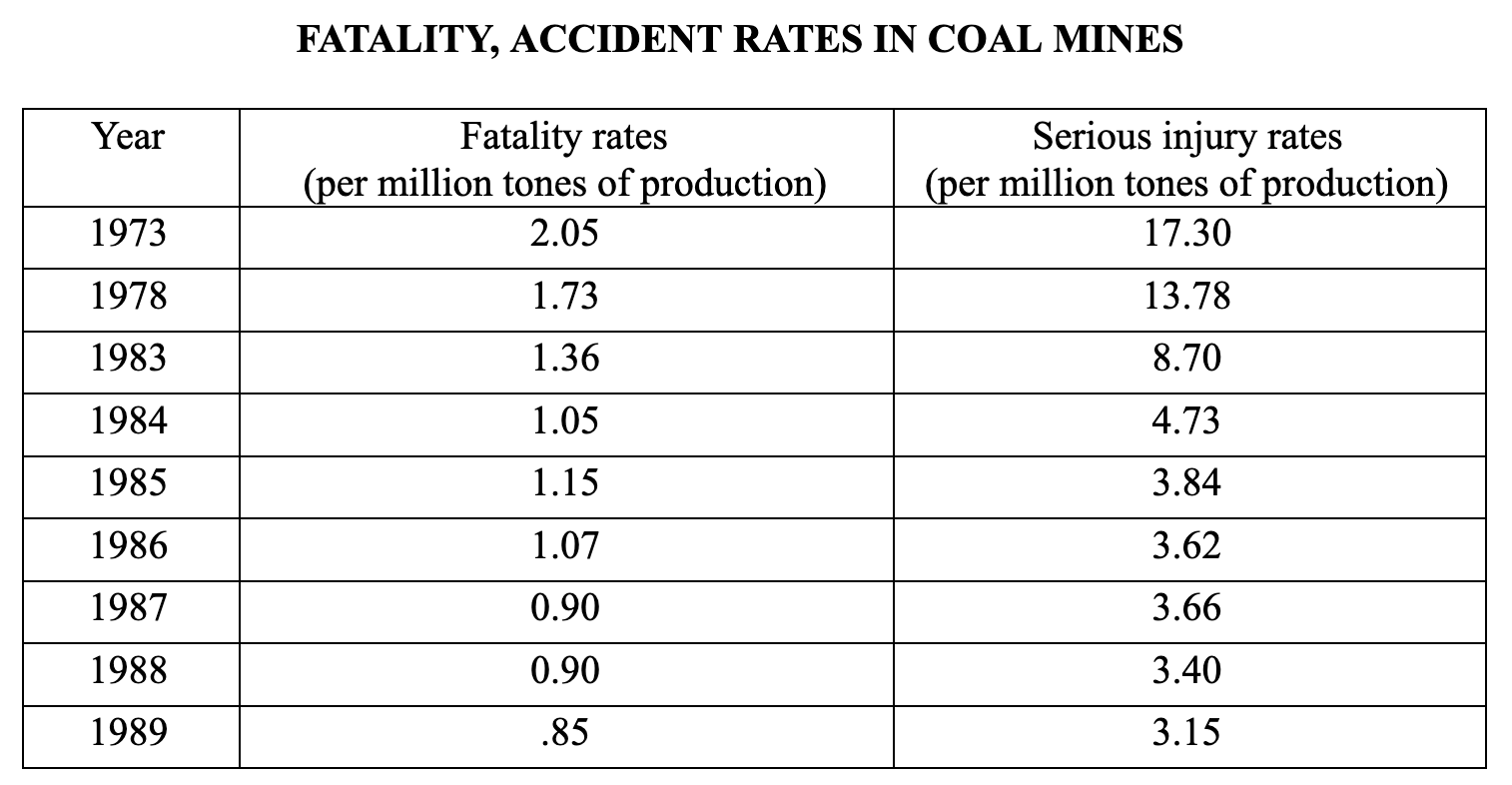If the total production of coal in 1989 was 225 million tones, what was the total number people who either died or were seriously injured in that year?
+

Explanation: Number of fatalities + Number of seriously injured = (0.85 +3.15) x 2.25 =900

Q #4
:

#### Quants Question

Directions: These questions are based on the following graph which shows the financial summary of company XYZ. The following definition are valid for the given data.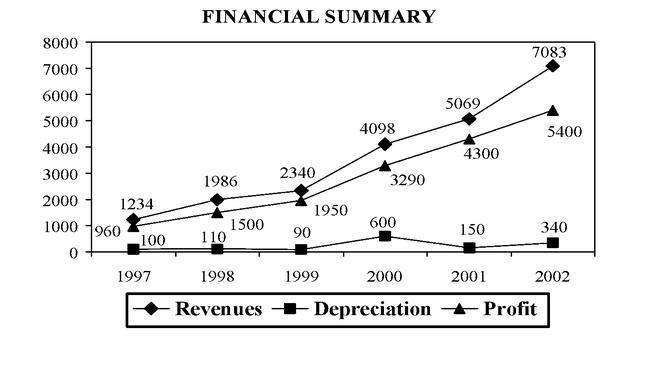• Profit Margin is defined as the ratio of profit to revenue
• Tax Margin is defined as (Revenue-Depreciation-Profit)/Revenue
• Depreciation Margin is defined as Depreciation/Profit

In which year did the company XYZ experience the highest profit margin?
+

Explanation : Profit margin is defined as the ratio of profit to revenue and it will be the highest when the profit is as close to Revenue as possible. By simple observation we can see that profit is closest to revenue in the year 1999 and 2001 out of the year 1999, 2000, 2001 and 2002.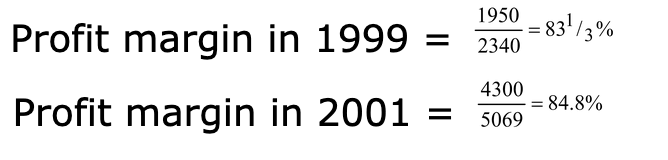Q #5
:

#### Quants Question

DIRECTIONS: are based on the following graph: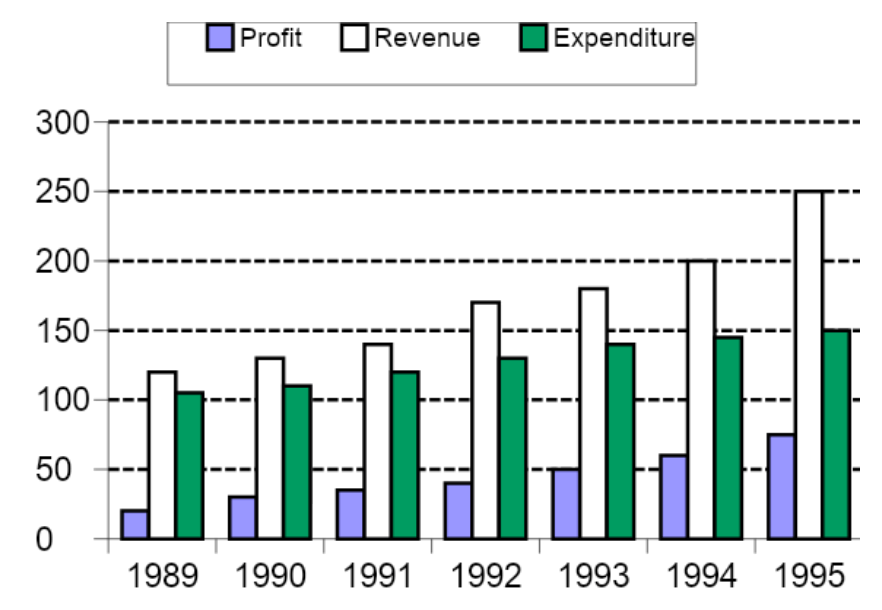Which year of showed the greatest percentage increase in profit as compared to the previous year ?
+

Explanation: Percentage Increase = [Final value - Initial value] 100/Initial value. Using this formula & conversion of fractions into percentage, calculate the percentage increase for the various years. Maximum percentage increase is for the year 1992 = (40 - 30)/30 = 1/3 = 33.33%.